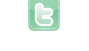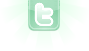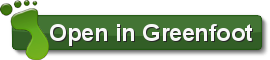Report as inappropriate.

# Simple Pendulum Simulation

Someday, I had to show for second time, now to Brawijaya Physics's students what I was knowed about Pendulum simulation. Startly that goes be well and I not have problem anything about coding. Then, when we want to enter the theoretically physics concept to Pendulum....ha.. ha :D, I was forgeted about that method and our work can't be completed. After that, I looking to the reference that explained this method for second time and finally this is our simulation. How Great God that created human to need each other not only once?

4321 views / 10 in the last 7 days

2 votes | 0 in the last 7 daysYour browser does not support the canvas tag.
Yeah, its just finding away to incorporate that into the code as I don't know java very well :/
Simple way is to add a variable called gravity that = 1. Then every time it reaches the highest point of which it can go the amount of force applied for it to go back around the other way is reduced by the amount of gravity there is.
Or another way of doing it which will make the gravity change as it moves up and down would go something like this. initiate a variable called gravity that = 0. have a check in the act that will make the gravity be equal to : (length of wire - distance between y coordinates of center point and the ball) / 10. Then make a check in the area of code where it applies the force which will do : If the ball is going downwards (Dipping down in the U shape) the force applied will + the amount of gravity there is. Otherwise if the ball is going upwards the force applied will - the amount of gravity.
By the way i do not understand what your code is saying that is why i am not giving you a fully formated piece of code to you, what i have given you is based on the information i can see from watching what the scenario is doing.
I would like it (if you haven't already) if you implemented the equation for the period of a pedulum: that is 2*PI*sqrt(Length of string (in meters)/9.8 meters per sec)
That is all in meters and assuming you are doing this on earth: so if you want to change it to your own variables, the basic equation is this: 2*PI*sqrt(length of pendulum/Gravitational acceleration)
That's the problem I face.. I don't know the math or have time to figure it out.
Once the ball moves, it doesn't stop anymore. Not so realistic.
Is there anyone that can look at this code, try and understand it, and then suggest ways of incorporating gravity so the pendulum will slow down to a stop, rather than continuously?